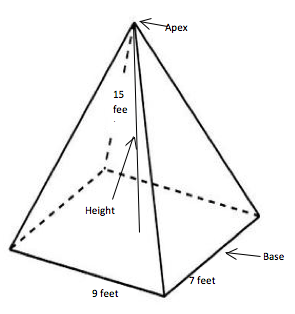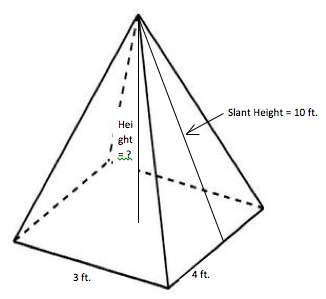Once you enter the world of three-dimensional shapes, math begins to take on a whole new layer of depth. Finding the volume of simple, ordinary shapes can be challenging for some, but when you start building upward and outward on those, the process becomes even more complicated. Pyramids happen to be among the most widely known 3D figures. Though you’ll find numerous types with any number of triangular faces, we’ll focus on rectangular pyramids for the time being.

Finding the Volume of a Rectangular Pyramid

Rectangular pyramids have four-sided bases and four triangular sides coming together in an apex, or what we know more simply as the pointy tip. Your overall formula for finding the volume of these multi-faceted shapes is V = (l x w x h) / 3. Basically, your first step is finding the area of the base by multiplying length by width.

Once you determine the area of the base, multiply that by the height. Height is the distance from the center point of the base to the apex. After multiplying the area of the base by the height, you’ll divide your answer by three to get the volume. Here’s an example of a rectangular pyramid and some sample measurements:Start off with your basic formula, plug in the given measurements and proceed with caution.

V = (l x w x h) / 3

V = (9 x 7 x 15) / 3

V = (63 x 15) / 3

V = 945 / 3

V = 315 feet3

You might also see the formula written as: V = 1/3 Bh. This just means you’re multiplying the base by the height and dividing by three, which is the same process. Keep in mind, both work for square pyramids as well since squares are types of rectangles.

In some cases, you may need to find the height of your pyramid before you’re able to work out its volume. Let’s say you’re given the slant height of the pyramid, which is the distance from the apex to the center of one of the triangular faces. This makes things a little more difficult but far from impossible. Here’s an example:Keep in mind, the line representing the height runs from the apex to the center of the base and forms a 90 degree angle at the base of the pyramid. This makes the triangle created by the height, slant height and base a right one, so you can use the Pythagorean Theorem (a2 + b2 = c2) to find your missing number. Your hypotenuse, or “c” side, is the longest one, which is always directly across from the 90 degree angle in a right triangle.

We don’t yet know the height, or the “a” side. Since the base measurement to be used here is three feet, from center to slant height would be 1.5. This’ll be your “b” side. Here’s the process for finding our missing height:

a2 + b2 = c2

a2 + 1.52 = 102

a2 + 2.25 = 100

a2 + 2.25 – 2.25 = 100 – 2.25

a2 = 97.75

a ≈ 9.9

With the knowledge your pyramid’s height is almost equal to 9.9, you can proceed as usual to solve for its volume.

Given the measurements of the base and height of a rectangular pyramid, you can solve to find its volume. If you have its slant height but not the actual height, let Pythagoras help you out before you try to solve for “V”. Whether you’re growing your geometry skills or gearing up for a trip to Giza, you’ll be ready to find the volume of any rectangular pyramid in your path!